Announcements
Product Release Webinar: Qlik Insider airing December 6! REGISTER TODAY!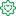Former Employee

## Recipe for a Pareto Analysis – Revisited

“Which products contribute to the first 80% of our turnover?”

This type of question is common in all types of business intelligence. I say “type of question” since it appears in many different forms: Sometimes it concerns products, but it can just as well concern any dimension, e.g. customer, supplier, sales person, etc. Further, here the question was about turnover, but it can just as well be e.g. number of support cases, or number of defect deliveries, etc.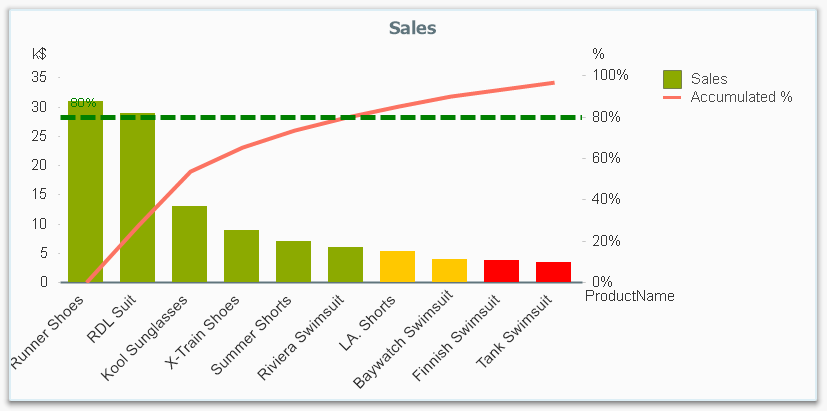It is called Pareto analysis or ABC analysis and I have already written a blog post on this topic. However, in the previous post I only explained how to create a measure which showed the Pareto class. I never showed how to create a dimension based on a Pareto classification – simply because it wasn’t possible.

But now it is.

But first things first. The logic for a Pareto analysis is that you first sort the products according to their sales numbers, then accumulate the numbers, and finally calculate the accumulated measure as a percentage of the total. The products contributing to the first 80% are your best, your “A” products. The next 10% are your “B” products, and the last 10% are your “C” products. In the above graph, these classes are shown as colors on the bars.

The previous post shows how this can be done in a chart measure using the Above() function. However, if you use the same logic, but instead inside a sorted Aggr() function, you can achieve the same thing without relying on the chart sort order. The sorted Aggr() function is a fairly recent innovation, and you can read more about it here.

The sorting is needed to calculate the proper accumulated percentages, which will give you the Pareto classes. So if you want to classify your products, the new expression to use is

=Aggr(
If(Rangesum(Above(Sum({1} Sales)/Sum({1} total Sales),1,RowNo()))<0.8, 'A',
If(Rangesum(Above(Sum({1} Sales)/Sum({1} total Sales),1,RowNo()))<0.9, 'B',
'C')),
(Product,(=Sum({1} Sales),Desc))
)

The first parameter of the Aggr() – the nested If()-functions – is in principle the same as the measure in the previous post. Look there for an explanation.

The second parameter of the Aggr(), the inner dimension, contains the magic of the sorted Aggr():

(Product,(=Sum({1} Sales),Desc))

This structured parameter specifies that the field Product should be used as dimension, and its values should be sorted descending according to Sum({1} Sales). Note the equals sign. This is necessary if you want to sort by expression.

So the Products inside the Aggr() will be sorted descending, and for each Product the accumulated relative sales in percent will be calculated, which in turn is used to determine the Pareto classes.

The set analysis {1} is necessary if you want the classification to be independent of the made selection. Without it, the classification will change every time the selection changes. But perhaps a better alternative is to use {\$<Product=>}. Then a selection in Product (or in the Pareto class itself) will not affect the classification, but all other selections will.

The expression can be used either as dimension in a chart, or in a list box. Below I have used the Pareto class as first dimension in a pivot table.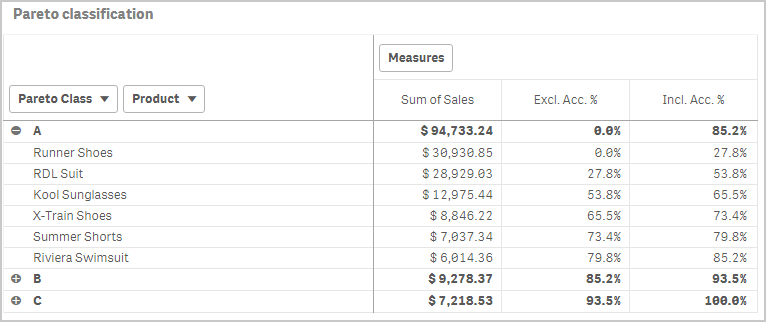If you use this expression in a list box, you can directly select the Pareto class you want to look at.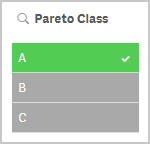The other measures in the pivot table are the exclusive and inclusive accumulated relative sales, respectively. I.e. the lower and upper bounds of the product sales share:

Exclusive accumulated relative sales (lower bound):

=Min(Aggr(
Rangesum(Above(Sum({1} Sales)/Sum({1} total Sales),1,RowNo())),
(Product,(=Sum({1} Sales),Desc))
))

Inclusive accumulated relative sales (upper bound):

=Max(Aggr(
Rangesum(Above(Sum({1} Sales)/Sum({1} total Sales),0,RowNo())),
(Product,(=Sum({1} Sales),Desc))
))

Good luck in creating your Pareto dimension!

HIC

Further reading related to this topic:

The sortable Aggr function is finally here!

Recipe for a Pareto Analysis

Recipe for an ABC Analysis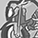Contributor III

thank you so much . It is working now

846 ViewsContributor III

Hi,

After changing the formula as suggested here, I am getting the output but still there are some values are being missed while it doing aggregation on it.

I am counting distinct Parent_supplier_id here  on basis of there spend. But it is missing some supplier id, even when it has <0.8 rangesum.

I am using below calculation, Please suggest if I am missing anything in here.

count(distinct aggr(if(RangeSum(Above(SUM({<\$(vSet,GP_IN_COUNTRY = {'Y'}>}SPEND_ACTUAL), 0, RowNo()))/
sum( {<\$(vSet),GP_IN_COUNTRY = {'Y'}>} TOTAL SPEND_ACTUAL)<=0.80,PARENT_SUPPLIER),
(PARENT_SUPPLIER,(=SUM({<\$(vSet),GP_IN_COUNTRY = {'Y'}>}SPEND_ACTUAL),Desc))))

849 ViewsPartner - Contributor III

Hi.

1. 'Above(SUM({<\$(vSet,' must be 'Above(SUM({<\$(vSet),'

2. 'count(distinct' must be 'count( {<\$(vSet),GP_IN_COUNTRY = {'Y'}>} distinct'

3. are you shure that the PARENT_SUPPLIER exactly the same as Parent_supplier_id ?

841 ViewsContributor III

hi ,

thank you for your response, I broken down the calculation further more to check where it is creating problem and removed variable value itself for test,  I used below calculation:

aggr(RangeSum(Above(SUM({<Period = {'2019-Q1','2019-Q2','2019-Q3'},[Period Key]=, GP_IN_COUNTRY = {'Y'}>}SPEND_ACTUAL), 0, RowNo())),
(PARENT_SUPPLIER_ID,(=sum({<Period = {'2019-Q1','2019-Q2','2019-Q3'},[Period Key]=,GP_IN_COUNTRY = {'Y'}>}SPEND_ACTUAL),desc)))

If you see in below screen shot, it is adding the spend value "A168899164671" in accumulation but it is not displaying the value for it.  the issue is , where ever it is missing to display those suppliers are not being consider in count and creates mismatch833 ViewsPartner - Contributor III

Hello All,
I am new to Qlikview and I am working on 80-20(Pareto) Analysis and Quadrant map in QlikView version 11.

I replicated the below link which explains it very well and my 80-20 chart for Customers and Products works fine

https://community.qlik.com/t5/Qlik-Design-Blog/Recipe-for-a-Pareto-Analysis/ba-p/1468497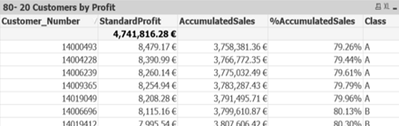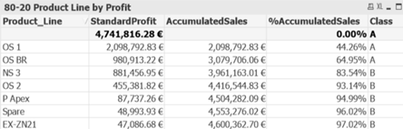Now, I am trying to match Customer_Number from Straight Table 1 and Product_Line from Straight Table 2 based on its Class and create four Quadrants that shows number of Customers & products from Class AA, Class AB, Class BA, and Class BB

I followed the advice from @hic  but unfortunately my Qlikview version does not support the expression below.

Customers:

=Aggr(If(Rangesum(Above(Sum({1} Standard_Profit)/Sum({1} total Standard_Profit),1,RowNo()))<=0.8, 'A','B'),
(Customer_Number,(=Sum({1}Standard_Profit),Desc)))

Products:

=Aggr(If(Rangesum(Above(Sum({1} Standard_Profit)/Sum({1} total Standard_Profit),1,RowNo()))<=0.8, 'A','B'),
(Product_Line,(=Sum({1}Standard_Profit),Desc)))

To Join the classes between Customers and Products:

=Aggr(NODISTINCT If(Rangesum(Above(Sum({1} Standard_Profit)/Sum({1} total Standard_Profit),1,RowNo()))<=0.8, 'A','B'),
(Customer_Number,(=Sum({1}Standard_Profit),Desc)))

&

=Aggr(NODISTINCT If(Rangesum(Above(Sum({1} Standard_Profit)/Sum({1} total Standard_Profit),1,RowNo()))<=0.8, 'A','B'),
(Product_Line,(=Sum({1}Standard_Profit),Desc)))

Now, I must group the classes AA, AB, BA, BB and create four lists that shows -

Quadrant 1: List of Customer Numbers with class 'A' from Straight Table 1 that has Product Line Class 'A' from straight table 2. Eg., Customer_Numbers in class A that has Product_Line in class A

Quadrant 2: List of Customer Number with class 'A' from Straight Table 1 that has Product Line Class 'B' from straight table 2. Eg., Customer_Numbers in class A that has Product_Line in class B

Quadrant 3: List of Customer Number with class 'B' from Straight Table 1 that has Product Line Class 'A' from straight table 2 Eg., Customer_Numbers in class B that has Product_Line in class A

Quadrant 4: List of Customer Number with class 'B' from Straight Table 1 that has Product Line Class 'B' from straight table 2. Eg., Customer_Numbers in class B that has Product_Line in class B

Can anyone please help me whether there is any other option to do so in QV 11 as unfortunately my company cannot upgrade to 12? It is very important for my project work and a little urgent one. I would highly appreciate your help. I have attached my file for your reference Thanks a lot, in advance.

782 ViewsContributor

je viens de tester,
le tri n'est pas pris en compte

677 ViewsContributor

Hi all,

I'm quite new to Qlik and I find a little bit hard moving away from the excel logic.

I have an issue that I'm not able to fix, if you have already answered to this type of question please discard my post.

I have a pivot with 3 fields: market, model and amount

model is sold in multiple markets

I created the Parteto field as per the above (no issue on this)

Now, how can I sort within the market the lines by model? How to setup the Aggr fuction?

I attached a schreenshot,  Ypsilon is the most sold model within the database but under Kenya is not (it should be listed at the third place)

thanks a lot Davideok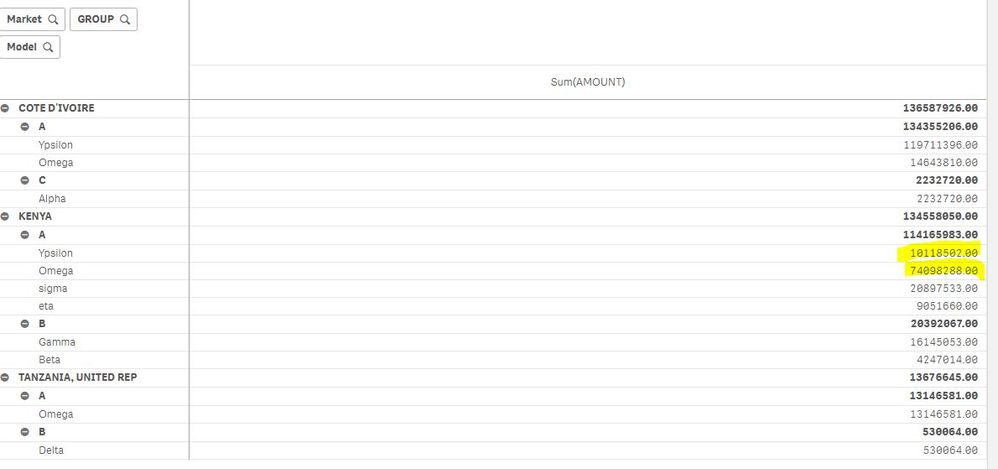551 ViewsPartner - Contributor III

Hi,

I am trying to make a pareto coloring in a table chart.

It drops error when I exit from edit mode....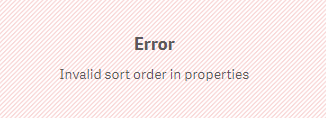although it works fine in edit mode...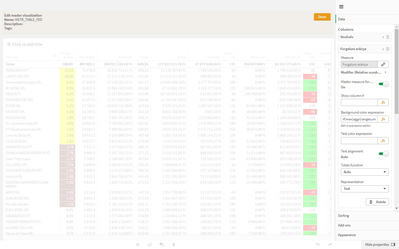Do you have any idea why?

Thanks and best regards,

Levente

390 Views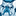Partner - Contributor III

Hello sir. I have some problems. My purpose is to ensure that the selection are independent. So I want the choices not to affect the values in the analysis. How do I do this?

220 Views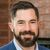Partner - Contributor II

@senerustunerciyas  you should write the Measure expression like this:

=Sum(

{<

[FieldWithSelection]=

>}

[ValueField])

This is using Set Analaysis to ignore a selection. Hope this helps!

203 Views
Subscribe by Topic